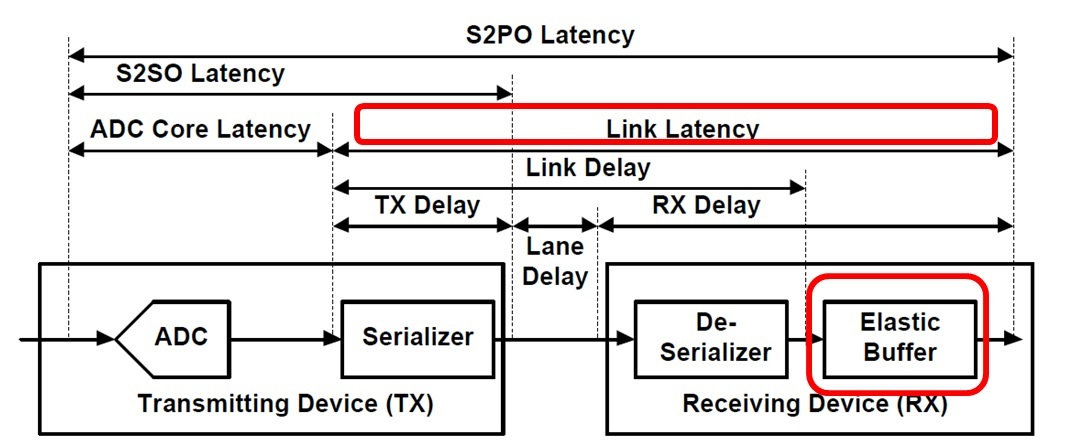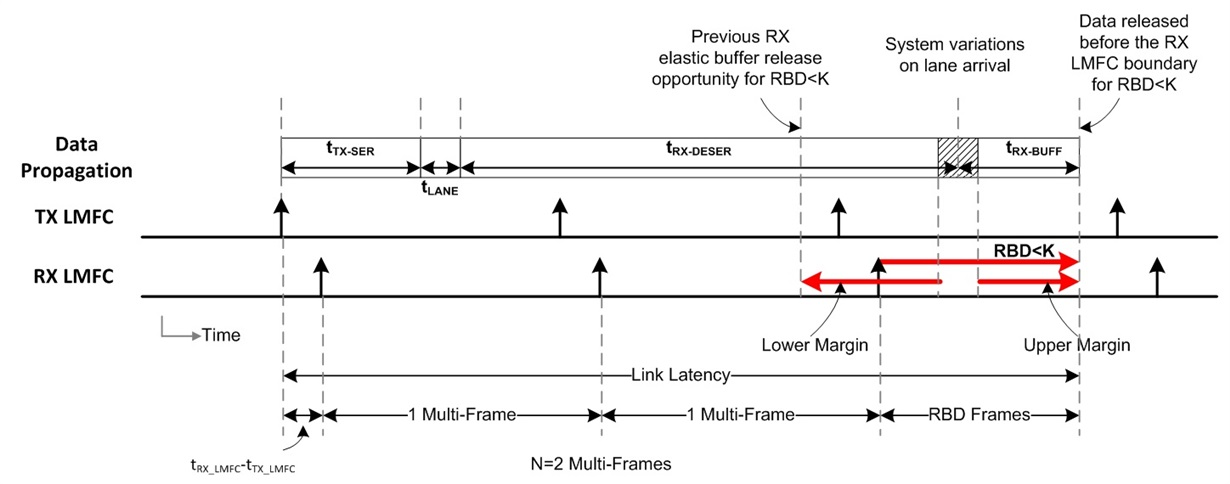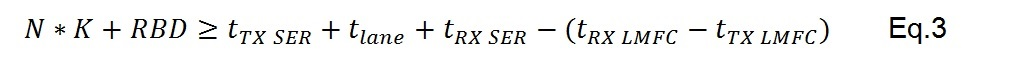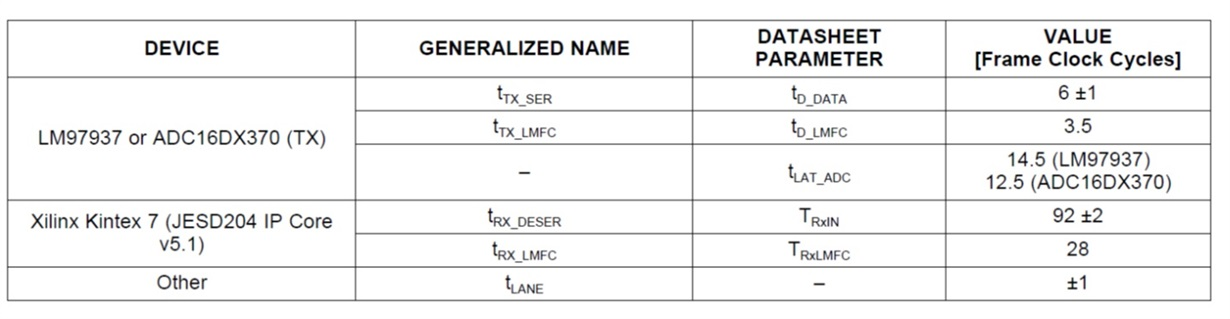# JESD204B: How to calculate your deterministic latency

In my previous blog, I explained how to achieve deterministic latency by aligning the LMFC signals at the transmit (TX) and receive (RX) devices and using the release buffer delay (RBD) to set the data release point to follow the expected arrival of the latest arriving lane. In this post, I will show you how to calculate the expected link latency using device parameters of the TX and RX devices.

Total latency is the sum of the analog-to-digital converter (ADC) core latency plus the link latency. ADC core latency can usually be found in the ADC datasheet. Link latency is defined as the time when samples enter the TX serializer, traverse the SERDES lanes, go through the RX de-serializer and come out of the elastic buffer. This is shown in figure 1.Figure 1. Summary of the total latency from signal input to parallel out (S2PO). It is comprised of the ADC core latency and the JESD204B link latency. You can adjust the elastic buffer to optimize link latency.

You can calculate the link latency using the following information, which should be available from the TX and RX device vendor:

• Determine the alignment of the TX and RX local multi-frame clock (LMFC) with respect to the arrival of SYSREF (subclass 1). Any offset between the TX and RX LMFC will be accounted for as a fixed delay. The device datasheet for the TX and RX typically provides this parameter as some number of frame clock cycles. The difference is given by tRX_LMFC-tTX_LMFC.
• Calculate the expected link delay accounting for system variations. The link delay starts at the TX LMFC edge and ends at the RX de-serializer output. The link delay is the sum of the TX serializer delay  tTX SER, the lane delay tlane and the RX de-serializer delay tRX SER.
• Choose the elastic buffer release point that provides margin against delay variations. Typically, the elastic buffer release point is set at the next LMFC edge following the arrival of the last lane. In this case, the RBD is set by default to K frames (1 MultiFrame) from the prior LMFC edge. This causes the data to release at the next LMFC edge following the arrival of the last lane. However, if all the lanes, including system variations, arrive at some point in between LMFC boundaries, the RBD can be set less than K to optimize the link latency.Figure 2. Link latency starts from the TX LMFC to when the data is released from the Elastic buffer. RBK<K can be used to optimize the link latency.

Once you’ve done this, you can calculate the link latency as the composite of the delays from the TX LMFC edge when the data enters the TX serializer to when the data comes out of the Elastic buffer. This includes the difference between the device TX-RX LMFC edges, some integer number of multi-frames spanning the link delay and the RBD number of frames in the elastic buffer. The total latency would then be the fixed ADC core latency + the link latency.  This can be expressed as a function of the frame cycle in the following equations:N = Minimum integer number of whole RX multi-frames spanning the link delay

K = Number of frames in a multi-Frame

RBD = Number of frames in the elastic buffer, worse case latency assume RBD=K

You can determine parameter N by meeting the requirements of the following equation, which states that the link delay (TX serializer delay + lane delay + RX serializer delay) minus the TX-RX delay must fit within the time span of N whole RX multi-frames + RBD frames of the elastic buffer:As an example, let’s look at Figure 3, which gives the parameters for the LM97937 ADC and Kintex 7 FPGA with K=32.Figure 3. Example link delay parameters for the LM97937 and Kintex 7 FPGA

For RBD set less than K (assume RBD=24), Eq.3 results in the following inequality:The minimum integer solution is given by N=2 and the resulting the link Latency (Eq.1) and total latency (Eq.2) would be:Check back in February for my next blog post, which will examine how to choose the RBD value and a method to measure and verify the calculated total latency.